# Invited Speakers

Maria Chudnovsky, Princeton, USA (Tuesday)
Coloring square-free perfect graphs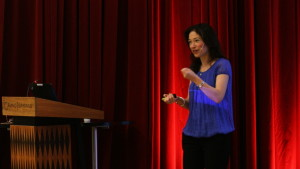Perfect graphs are a class of graphs that behave particularly well with respect to coloring. In the 1960’s Claude Berge made two conjectures about this class of graphs, that motivated a great deal of research in the field in the second half of the 20th century. One of the conjectures, The Weak Perfect Graph Conjecture, was solved by Laszlo Lovasz in 1972, the other, The Strong Perfect Graph Conjecture, by Robertson, Seymour, Thomas and the speaker in the early 2000’s.
The following remained open however: design a combinatorial algorithm that produces an optimal coloring of a perfect graph. Recently, in joint work with Lo, Maffray, Trotignon and Vuskovic we were able to construct such an algorithm under the additional assumption that the input graph is square-free (contains no induced four-cycle). Historically, the class of square-free perfect graphs seems to be a good special case to look at; in particular, this was the last special case of the Strong Perfect Graph Conjecture that was solved before the general one.

Amin Coja-Oghlan, Goethe University, Germany (Thursday)
Random Graph Coloring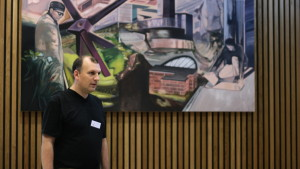Consider a random graph G(n,m) and an integer k>2. For what values of m/n is G(n,m) k-colorable? If it is k-colorable, how many k-colorings does it have, and what are the geometric and probabilistic properties of the set of k-colorings. In this talk I am going to give an overview of recent work on this subject.

Zdenek Dvorak, Charles University, Prague (Friday)
Detailed structure of embedded 4-critical triangle-free graphsBy a well-known theorem of Grötzsch, every triangle-free planar graph is 3-colorable. This result has been extended in many ways, especially for graphs embedded in other surfaces. In this talk, we explore the following question: given a triangle-free graph G embedded in the plane (or another surface) and a set S of vertices of G, which 3-colorings of S extend to a 3-coloring of G?

Algorithmically, this question was solved by Dvorak, Kral and Thomas (2009). However, we are more interested in the structural aspects: can G be decomposed into a bounded number of well-behaved pieces which determine the set of extendable colorings of S?

We present a positive answer to this question which we obtained in a joint work with Bernard Lidicky. To give a full description of the structure, we combine results obtained using several recently developed methods (weight-increasing reductions, potential method, topological methods) with computer-aided enumeration.

Pavol Hell, Simon Fraser University, Canada (Friday)
Forbidden structure characterizations of interval and circular arc graphs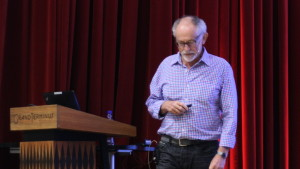I will describe a novel forbidden structure characterization of interval graphs with implications for interval digraphs and bigraphs. I will also discuss a related characterization of circular arc graphs. This is the first forbidden structure characterization of circular arc graphs, and it implies a polynomial time certifying algorithm. Joint with T. Feder, J. Huang, and A. Rafiey for the interval graphs and generalizations, and with M. Francis and J. Stacho for the circular arc graphs.

Daniel Lokshtanov, University of Bergen, Norway (Monday)
Graph Modification Problems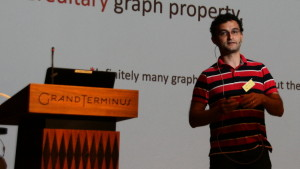In a vertex deletion problem the input is a graph G, and the task is to delete a smallest possible set S of vertices such that the resulting graph has a certain property. Many of the well-known NP-complete optimization problems are (or can be re-stated as) vertex deletion problems. For an example, the Vertex Cover problem is simply to find the smallest vertex set S such that G – S has no edges. In the Feedback Vertex Set problem the task is to find a smallest vertex set S such that G – S is acyclic. Most vertex deletion problems are NP-hard. In this talk we will survey some of the approaches to deal with the NP-hardness of vertex deletion problems, including approximation algorithms, parameterized algorithms and kernelization, as well as the combinatorial insights behind the algorithmic results.

Francisco Santos, Universidad de Cantabria, Spain (Thursday)
Enumerating lattice 3-polytopes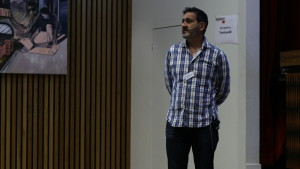A lattice 3-polytope is a polytope P with integer vertices. We call size of P the number of lattice points it contains, and width of P the minimum, over all integer linear functionals f, of the length of the interval f(P). For example, P has width one if it is contained between two consecutive parallel lattice planes.
We are interested in the classification of lattice 3-polytopes modulo affine integer isomorphism, which we call unimodular equivalence.
Lattice 3-polytopes of size four (that is, “empty lattice tetrahedra”) are classified since long, and they all are known to have width one. However, there are infinitely many of them, which implies that there are also infinitely many 3-polytopes of any given size greater than four. However, we show that all but finitely many of them have width one, which suggests the possibility of completely classifying those of width larger than one. In this talk we report on an algorithm to do this,
For sizes five and six our methods are quite ad-hoc, combining geometric and oriented matroid techniques with a detailed case study of the possible configurations that arise. Starting with size seven we use a more structured approach, based on the proof that every lattice 3-polytope of width larger than one falls into one of the following three categories:

1. It has (at least) two vertices u and v whose removal still has width larger than one. Polytopes of this type can be all obtained “gluing” smaller polytopes of width larger than one.
2. It projects orthogonally to one of a list of five particular 2-polytopes of size at most six. 3-polytopes of this type can be described explicitly, for each size.
3. It is what we call “boxed”: except for three of its lattice points, P is contained in the unit cube. Boxed 3-polytopes have size at most 10 and we have completely enumerated them with the help of computer (there are 23 of size 7, 7 of size 8, and only one of sizes 9 and 10).

Our computations are still ongoing but we now have a list of 493 3-polytopes of size seven and width larger than one, that we believe to be complete. Part of our motivation is to answer certain questions of Reznick et al. concerning “distinct-pair-sum” 3-polytopes, which are known to have size at most eight.
This is joint work with Monica Blanco.

Van Vu, Yale University, USA (Monday)
Anti-concentration Inequalities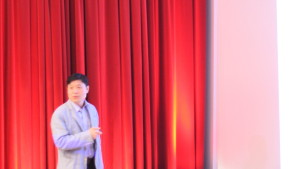In probability, one often needs to show that a (bad) event holds with very small probability.
In many cases, the event takes the form of a real random variable X belongs to an interval I.
The anti-concentration phenomenon asserts that (under certain assumptions) the probability that X taking value in a short interval I is small. For instance, if X is the sum of n iid {-1,1} random variables, then the probability that it belongs to any interval of length 1 is at most C n. The first general anti-concentration inequality was discovered by Littlewood and Offord in the 1940s, with extensions by Erdos, Esseen, Kolmogorov, Rogozin,
Kleitman, Halasz and many others.
In the last ten years or so, the field has been revitalized, fueled by studies in random structures and theoretical computer science. As a result, we have now obtained a near complete understanding of the anti-concentration phenomenon in the case when X is a linear function of independent random variables. This understanding plays an essential role in the establishment of various long standing problems in totally unrelated areas.
This talk is aimed as a survey towards a general audience, focusing on a few main ideas and applications, with many open problems.

Imre Barany (Tverberg session), Alfréd Rényi Mathematical Institute of the Hungarian Academy of Sciences, Hungary (Wednesday)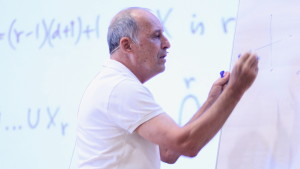Günter M. Ziegler (Tverberg session), Freie Universität, Germany (Wednesday)